Next: 4.7 Discussion Up: 4. Abstract recurrent neural Previous: 4.5.2 Results

# 4.6 Dependence on the number of input dimensions

As shown in section 4.5, the performance of the abstract recurrent neural network depends on the number of input dimensions chosen. A higher number of input dimensions results in a higher sum of square errors per pattern. This relationship is investigated theoretically on a simplified abstract RNN.

The first simplification is that the model consists of spheres instead of ellipsoids. Thus, the distribution of training data is approximated by a set of m code-book vectors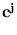, and for each of them the potential field is given by the Euclidean distance. The second simplification is that the code-book vectors are uniformly randomly distributed. This is justified for distributions that are wrapped inside the pattern space, and are not restricted to embedded hyper-planes, as it is likely the case for the kinematic arm model. Recall works as in section 4.2 (see also figure 4.14).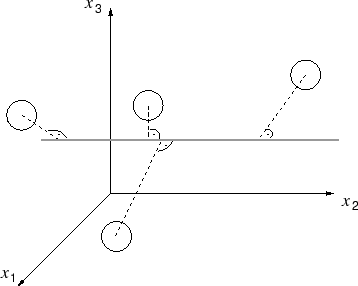We assume that the code-book vectors lie inside a d-dimensional cube of side length two, centered at the origin. Since the code-book vectors are distributed uniformly, the average error is independent of the specific value of the input (offset of the constrained space)4.3. Thus, we arrange the constrained spaces such that they all go through the origin. If d - 1 input dimensions are given, the constraint is the xd-axis. For d - 2 input dimensions the axis xd and xd-1 span the constraint space, and so on (see figure 4.15 as an example). Instead of choosing different input values to compute the sum of square errors, we draw a new set offrom a random distribution for each test trial.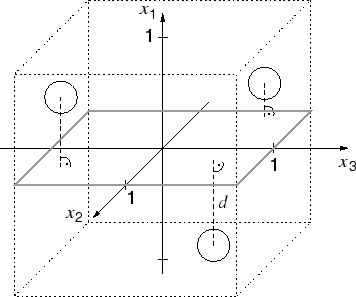Given r input dimensions, the squared distance Ej ofto the constrained space is

 Ej =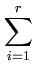c ji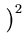. (4.8)

We define the square error E as the minimum squared distance to the data approximation (in the kinematic arm model, this matches the computation of the square error in the case of arbitrary directions, see section 4.5.1). Thus,

 E = min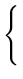(c1i)2,(c2i)2,...,(cmi)2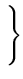. (4.9)

The sum of square errors per pattern is the expectation value of E given a random distribution of. To compute the expectation of a minimum, we use the following trick (Wentzell, 2003). The cumulative probability Pc(T) that all Ej are larger than a threshold T is computed. Pc(T) is monotone descending, starting with Pc(0) = 1. The negative derivative of Pc(T) can be interpreted as the probability density function of T. For a given T, this function provides the probability density that T equals the smallest member of the set {Ej}. Thus, the expectation value of T (the first momentum of the probability density function) equals the expectation value of E, the minimum of {Ej}. Therefore,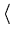E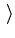=T=   -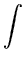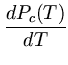T dT . (4.10)

The probability p that a pointhas a squared distance Ej larger or equal to T is the cube volume outside the r-sphere4.4with radiuscentered at the origin divided by the cube volume (figure 4.16). The cumulative probability Pc is p to the power of m.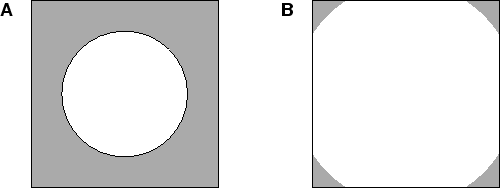To make the function p over T analytically integrable, we make an approximation. The r-cube, the space of all possible codebook vectors (in the first r dimensions), is replaced by an r-sphere with radius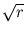centered at the origin. This sphere encloses tightly the r-cube. To compensate this step, we multiply the number of codebook vectors m with the r-sphere volume divided by the r-cube volume. The volume of an r-sphere with unit radius can be written as

 Vr =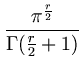. (4.11)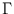is the Gamma-function. It is related to the factorial by(n) = (n - 1)! for positive integers n. For r = 2, for example, the above volume Vr equals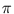. Hence, the resulting volume relation vr (here, for the sphere radius) is

 vr =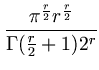. (4.12)

The above step is justified by the uniform distribution of the. But, it is not an equivalence transformation (for r > 1). The quality of this approximation will be tested later. The resulting number of vectors,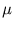= int(vrm), is rounded to an integer value. With the approximation, the probability p(T) that a vector has a squared distance Ej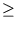T can be expressed as

 p(T) = 1 -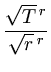. (4.13)

The total probability Pc that all vectors fulfill the above condition is

 Pc(T) =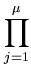p(T) = (1 - (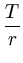)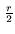)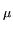. (4.14)

The value of T extends from 0 to r. Using (4.10), we obtain for the expectation value of EE=   -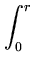T dT =Pc(T)dT - Pc(T)T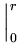=Pc(T)dT . (4.15)

The second equality sign uses integration by parts, the last equality sign uses (4.14). The final integration, using (4.14), givesE= r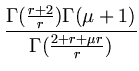. (4.16)

The integral was solved with the help of MATLAB® and its symbolic toolbox. Using the equality(x) = (x - 1)(x - 1), the expression can be simplified toE= r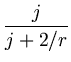. (4.17)

For large, the latter is more feasible for numerical evaluation than (4.16). We further investigate the quality of our approximation. The case m = 1 can be evaluated correctly. For one codebook vector, the expectation of its squared distance can be directly calculated:E= 2-r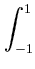...ci2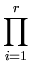dci = 2-rr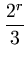=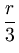. (4.18)

Figure 4.17 shows the result of this comparison. The mismatch increases with the number of input dimensions r.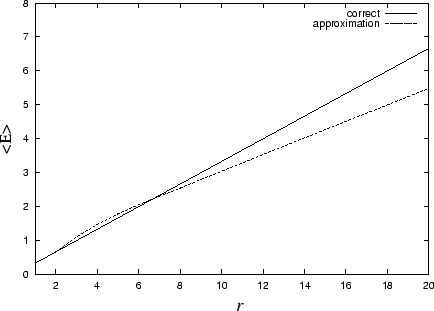We want to check our approximation using larger m. The result from (4.12) and (4.17) was compared to a simulation, in whichwere drawn randomly from a r-cube with side length 2. Figure 4.18 shows the result, using r = 10 input dimensions and 100 000 trials for each m value in the simulation. The approximation got better the more codebook vectors were used. The number of codebook vectors needed for a good approximation depends on the value of r. The higher r, the more vectors are needed.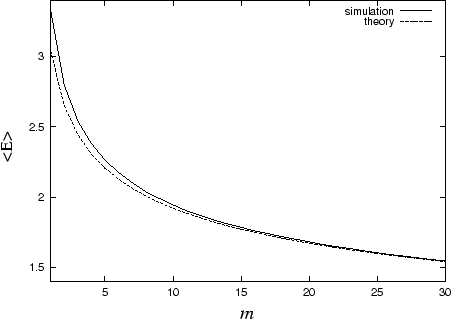Finally, the dependency on the number of input dimensions is demonstrated for the case m = 200. As above, the theory is compared to a simulation, using 100 000 trials for each r value. In this test, theory and simulation results did overlap (figure 4.19). Between r = 5 and r = 8 the increase is approximately exponential with exponent 0.69.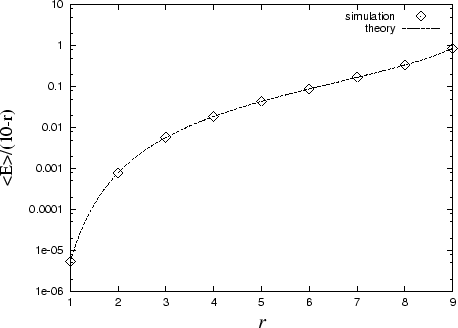Next: 4.7 Discussion Up: 4. Abstract recurrent neural Previous: 4.5.2 Results
Heiko Hoffmann
2005-03-22